Next: Isotropic Tensors Up: Cartesian Tensors Previous: Tensor Transformation

# Tensor Fields

We saw in Appendix A that a scalar field is a set of scalars associated with every point in space: for instance,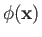, where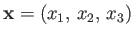is a position vector. We also saw that a vector field is a set of vectors associated with every point in space: for instance,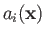. It stands to reason, then, that a tensor field is a set of tensors associated with every point in space: for instance,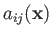. It immediately follows that a scalar field is a zeroth-order tensor field, and a vector field is a first-order tensor field.

Most tensor fields encountered in physics are smoothly varying and differentiable. Consider the first-order tensor field. The various partial derivatives of the components of this field with respect to the Cartesian coordinates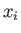are written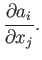(B.50)

Moreover, this set of derivatives transform as the components of a second-order tensor. In order to demonstrate this, we need the transformation rule for the, which is the same as that for a first-order tensor: that is,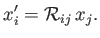(B.51)

Thus,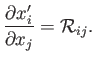(B.52)

It is also easily shown that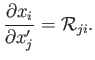(B.53)

Now,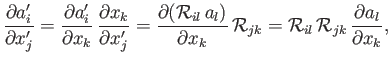(B.54)

which is the correct transformation rule for a second-order tensor. Here, use has been made of the chain rule, as well as Equation (B.53). [Note, from Equation (B.26), that the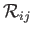are not functions of position.] It follows, from the previous argument, that differentiating a tensor field increases its order by one: for instance,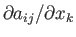is a third-order tensor. The only exception to this rule occurs when differentiation and contraction are combined. Thus,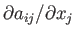is a first-order tensor, because it only contains a single free index.

The gradient (see Section A.18) of a scalar field is an example of a first-order tensor field (i.e., a vector field):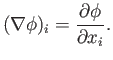(B.55)

The divergence (see Section A.20) of a vector field is a contracted second-order tensor field that transforms as a scalar: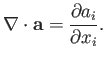(B.56)

Finally, the curl (see Section A.22) of a vector field is a contracted fifth-order tensor that transforms as a vector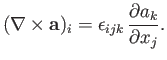(B.57)

The previous definitions can be used to prove a number of useful results. For instance,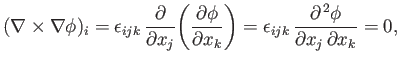(B.58)

which follows from symmetry because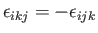whereas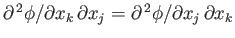. Likewise,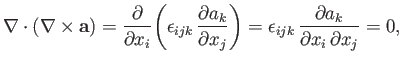(B.59)

which again follows from symmetry. As a final example,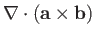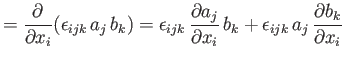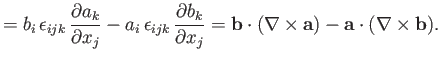(B.60)

According to the divergence theorem (see Section A.20),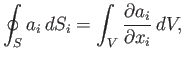(B.61)

whereis a closed surface surrounding the volume. The previous theorem is easily generalized to give, for example,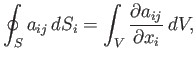(B.62)

or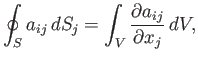(B.63)

or even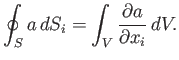(B.64)Next: Isotropic Tensors Up: Cartesian Tensors Previous: Tensor Transformation
Richard Fitzpatrick 2016-03-31